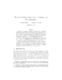## Modified Cholesky Algorithms: A Catalog with New Approaches##### Files
tr.pdf(423.01 KB)
Given an $n \times n$ symmetric possibly indefinite matrix $A$, a modified Cholesky algorithm computes a factorization of the positive definite matrix $A+E$, where $E$ is a correction matrix. Since the factorization is often used to compute a Newton-like downhill search direction for an optimization problem, the goals are to compute the modification without much additional cost and to keep $A+E$ well-conditioned and close to $A$. Gill, Murray and Wright introduced a stable algorithm, with a bound of $\|E\|_2=O(n2)$. An algorithm of Schnabel and Eskow further guarantees $\|E\|_2=O(n)$. We present variants that also ensure $\|E\|_2=O(n)$. Mor\'{e} and Sorensen and Cheng and Higham used the block $LBL^T$ factorization with blocks of order $1$ or $2$. Algorithms in this class have a worst-case cost $O(n3)$ higher than the standard Cholesky factorization, We present a new approach using an $LTL^T$ factorization, with $T$ tridiagonal, that guarantees a modification cost of at most $O(n2)$.